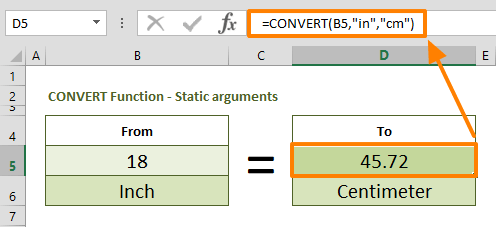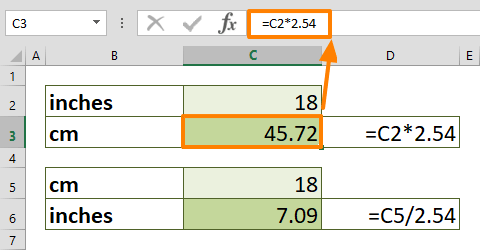Converting units can be time consuming when you rely on a separate tool or website to do it. In this guide, we are going to show you how to convert cm to inches and inches to cm in Excel.

## Manual approach

The first approach we are going to show you is doing the conversion manually. You can convert inches to cm using a simple math calculation. 1 inch is approximately equal to 2.54 cm. Thus, you need to multiply the centimeter-value by 2.54 to get the same value in inches.

Let's say the cell C2 contains the inch-value. Use a formula like below to find the inch-value.

=C2*2.54In a similar way, you can convert cm to inches. As shown in the above example, dividing a centimeter-value by 2.54 returns the value in inches. If we assume that the cell C5 contains the centimeter-value, the conversion formula would be

=C5/2.54

Now, let's take a look at more dynamic and relatively easy to remember approaches.

## Using the CONVERT Function

Another way to convert inches to cm is the CONVERT function. The CONVERT function can convert a number from one measurement system to another. The best part is that the function is not limited to only length units.  You can convert weight, distance, temperature, and more units of measurements between each other.

You can find more information about the CONVERT function with samples in our guide: Excel CONVERT Function

Briefly, you need to enter both the value and measurement types into the function. Excel accepts string abbreviations of the measurement types. In this example you can use,

• Inches: "in"
• Centimeters: "cm"

As a result, to convert the value in cell B5, you can use a formula like below:

=CONVERT(B5,"in","cm")To make the conversion in the opposite direction, simply switch the measurement arguments. The following formula converts the value from inches to cm.

=CONVERT(B5,"cm","in")## Another dynamic method

You can set cell references for unit arguments. This application allows you to control the from and to unit types. We combined the CONVERT and VLOOKUP functions to create a master formula which gets units to convert from a list or any other input type. With the help of the VLOOKUP function, we can use user-friendly names for unit type codes. For example, "centimeter" for "cm" and "inch" for "in".

The VLOOKUP function returns the corresponding unit type for the CONVERT function. Here is what the formula would look like:

=CONVERT(B11,VLOOKUP(B12,Length.Table,2,0),VLOOKUP(D12,Length.Table,2,0))

The cells B12 and D12 contain a dropdown list with the length type of measurement units like "Inch" and "Centimeter" strings. The VLOOKUP searches for these values in the named range Length.Table, and returns the corresponding value.